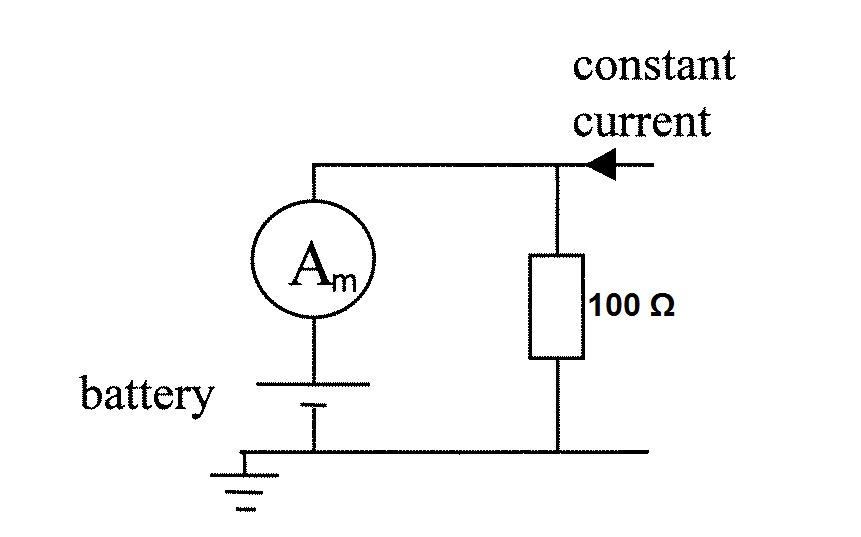#### You may also like### Overarch 2

Bricks are 20cm long and 10cm high. How high could an arch be built without mortar on a flat horizontal surface, to overhang by 1 metre? How big an overhang is it possible to make like this?### Stonehenge

Explain why, when moving heavy objects on rollers, the object moves twice as fast as the rollers. Try a similar experiment yourself.### Maximum Flow

Given the graph of a supply network and the maximum capacity for flow in each section find the maximum flow across the network.

# Battery Modelling

##### Age 16 to 18Challenge Level

A battery is modelled as a $24\mathrm{V}$ EMF in series with an internal resistance of $2\Omega$. The battery is being charged by a constant current source as shown in the network below. The ammeter, used to measure the current through the battery, has a voltage drop of $2\mathrm{V}$ at full range scales. Here it is set to a $20\mathrm{A}$ range and reads a current of $2\mathrm{A}$.

What is the value of the constant current supplied?

What fraction of the input power is supplied to the battery?

What current would flow into the battery if we now replaced the ammeter with a wire of zero impedance?